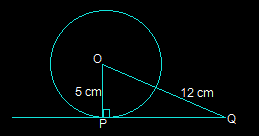# A tangent PQ at a point P of a circle of radius 5 cm meets a line through the centre O at a point Q so that OQ = 12 cm. Length PQ is : (A) 12 cm (B) 13 cm (C) 8.5 cm (D) √119 cm

Option D=√119From the above figure, the line that is drawn from the centre of the given circle to the tangent PQ is perpendicular to PQ.

Hence OP ⊥ PQ

Applyong Pythagoras theorem in triangle

ΔOPQ we get,

OQ2 = OP2+PQ2

(12)2 = 52+PQ2 PQ2

= 144-25 PQ2

= 119 PQ

= √119 cm

So, option D i.e. √119 cm is the length of PQ.